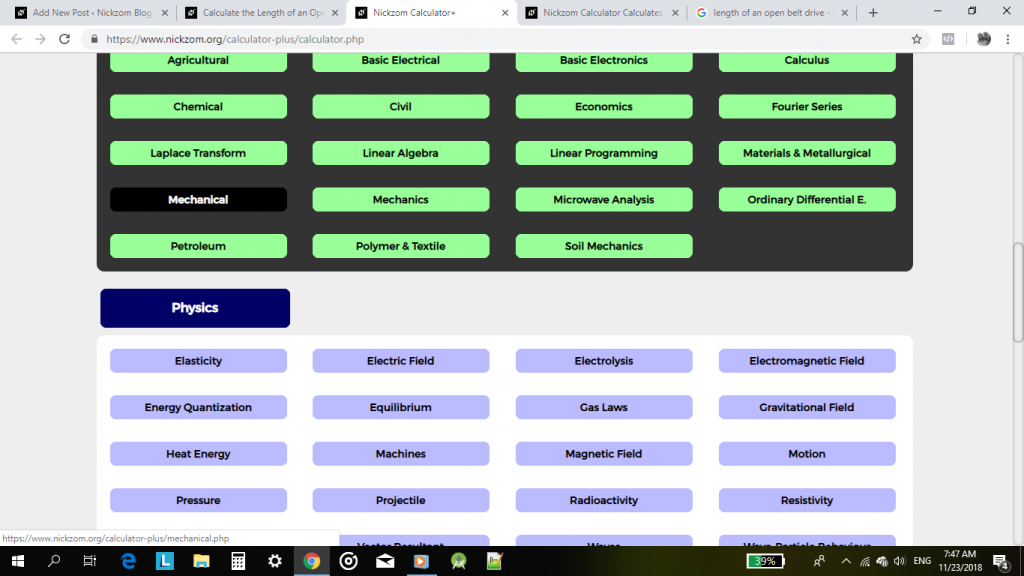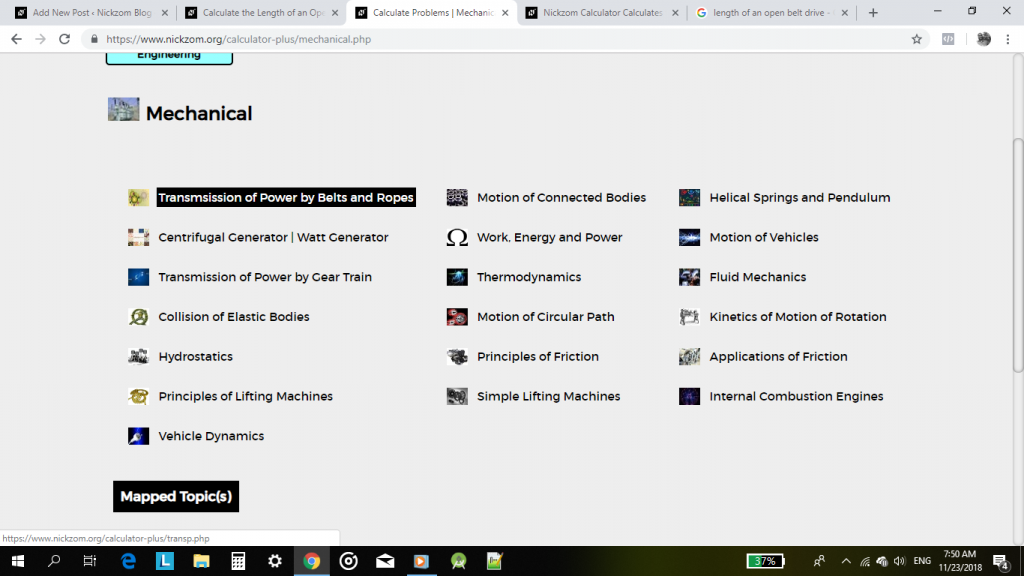## How to Calculate and Solve for Linear Expansivity, Area Expansivity and Volume or Cubic Expansivity

Linear expansivity, area expansivity and volume or cubic expansivity are all parameters that are being governed by heat energy. All three parameter is dependent on temperature rise which is primarily in Celsius unit of temperature.

Linear expansivity additionally depends on the original length and final length, area expansivity additionally depends on the original area and final area and lastly, cubic or volume expansivity depends on the original volume and final volume.

The formula for calculating linear expansivity is (l2 – l1) / l1θ
l2 represents the final length.
l1 represents the original length.
θ represents the temperature rise in Celsius.

The formula for calculating area expansivity is (A2 – A1) / A1θ
A2 represents the final area.
A1 represents the original area.
θ represents the temperature rise in Celsius.

The formula for calculating volume or cubic expansivity is (V2 – V1) / V1θ
V2 represents the final volume.
V1 represents the original volume.
θ represents the temperature rise in Celsius.

## The Calculator Encyclopedia Calculates the Length of An Open Belt Drive in the Transmission of Power by Belts and Ropes

Calculating the length of an open belt drive is a common thing in Mechanical Engineering. It was of those crucial parameters in the determining the transmission of power by belts and ropes.

In our world today, there are a lot of mechanical engines making use of belts and I believe as a Mechanical Engineer having access to Nickzom Calculator+ is one of the best tools to make lifer easier, faster and simpler.

Nickzom Calculator+ (Professional Version) – The Calculator Encyclopedia can solve over 10,000 calculations for a day to day Mechanical Engineer and today I would show you the steps on how to get the answer for the length of an open belt drive using Nickzom Calculator.

First and Foremost, you need to have access to Nickzom Calculator+ via any of these means:

You must also know that to get the length of an open belt drive there are 3 major parameters you need which are:

• Distance between the Centres of the Larger and Smaller Pullerys
• Radius of the Larger Pulley
• Radius of the Smaller Pulley

Once, you have access to the professional version of Nickzom Calculator via any means, move over to the calculator map, then scroll to the Engineering section and click on Mechanical.On clicking on Mechanical, you see a bunch of major topics that can be solved by Nickzom Calculator. Click on Transmission of Power by Belts and Ropes.